# Process Calculus

#### SinHarvest24

##### Shiroyasha
OP
Member
Ok, i started classes a few days ago and we did something called Process Calculus. We're learning how to make/change the subject of the equation. I'm a bit confused on a few things.

Say i have this: r - t * a/b = k (make "b" the subject, a/b being a fraction)

Answer : r - t * a/b = k
r * a/b = k + t
a/b = k + t - r
a = b(k + t - r)
a/k+t-r = b

Is that correct?

When i was doing it in class i was moving the wrong unknown first and ended up doing crap most of the time.

Any tips?

You can also give me a question, i like to practice.Member
r - t * a/b = k
rb - ta = kb
-ta = b(k-r)
b = - ta/(k-r)

Hope this helps.

#### Wizerzak

##### Because I'm a potato!
Member
sinharvest24 said:
Ok, i started classes a few days ago and we did something called Process Calculus. We're learning how to make/change the subject of the equation. I'm a bit confused on a few things.

Say i have this: r - t * a/b = k (make "b" the subject, a/b being a fraction)

Answer : r - t * a/b = k
r * a/b = k + t
a/b = k + t - r
a = b(k + t - r)
a/k+t-r = b

Is that correct?

When i was doing it in class i was moving the wrong unknown first and ended up doing crap most of the time.

Any tips?

You can also give me a question, i like to practice.I'm not 100% sure but i'm pretty certain that with the bit in bold you can't do that.... remember BIDMAS (or BODMAS w/e)

#### SinHarvest24

##### Shiroyasha
OP
Member
smealum said:
r - t * a/b = k
rb - ta = kb
-ta = b(k-r)
b = - ta/(k-r)

Hope this helps.
What did you do there? got rid of the fraction?
So what i did was entirely wrong?

Wizerzak said:
QUOTE(sinharvest24 @ May 24 2011, 06:34 PM) Ok, i started classes a few days ago and we did something called Process Calculus. We're learning how to make/change the subject of the equation. I'm a bit confused on a few things.

Say i have this: r - t * a/b = k (make "b" the subject, a/b being a fraction)

Answer : r - t * a/b = k
r * a/b = k + t
a/b = k + t - r
a = b(k + t - r)
a/k+t-r = b

Is that correct?

When i was doing it in class i was moving the wrong unknown first and ended up doing crap most of the time.

Any tips?

You can also give me a question, i like to practice.I'm not 100% sure but i'm pretty certain that with the bit in bold you can't do that.... remember BIDMAS (or BODMAS w/e)

Well, i'm just following the same steps i learned in class. Can anyone else confirm that i can't do that?
Do you agree with the first poster?

#### Wizerzak

##### Because I'm a potato!
Member
smealum said:
r - t * a/b = k
rb - ta = kb
-ta = b(k-r)
b = - ta/(k-r)

Hope this helps.

possibly right, i can't see how he did the bit in bold but it's possibly correct.

I recommend this site which gives free, online, live tutoring. I've used it a few times and the teachers there are great. Say Wizzerzak told you about it, they might remember me.

Member
r - t . a/b = k
r - ta/b = k
r = ta/b + k
r - k = ta/b

(r - k)b = ta

b = ta/(r - k)

#### Wizerzak

##### Because I'm a potato!
Member
Cuelhu said:
r - t . a/b = k
r - ta/b = k
r = ta/b + k
r - k = ta/b

(r - k)b = ta

b = ta/(r - k)

Yes, I see now. That's definitely right.

#### SinHarvest24

##### Shiroyasha
OP
Member
Cuelhu said:
r - t . a/b = k
r - ta/b = k
r = ta/b + k
r - k = ta/b

(r - k)b = ta

b = ta/(r - k)
like what you did there, can you give me some tips on when do i do those things. Which one do i move first and which not to.

#### The Pi

##### Lurker
Member
r - t * a/b = k Write it down first
rb - ta = kb Knock out any and all fractions
rb - kb = ta Move all terms containing the variable you're trying to solve for to the left and everything else to the right
b (r - k) = ta Single out the variable
b = ta / (r - k) Solve for the variable

Simples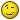#### SinHarvest24

##### Shiroyasha
OP
Member
Hit me a question/s lemme see if i got this.

#### Jamstruth

##### Secondary Feline Anthropomorph
Member
This isn't Calculus. This is algebra.

r- t * a/b = k
(r - t)(a/b) = k
(a(r - t))/b = k
a(r - t) = kb
(a(r - t))/k = b

In the way you wrote it it would be
(r - t) * (a/k)

I'm sure that's how its done if I'm reading the equation right. I much prefer using brackets than * symbols in these equations. The problem when you're making up things like this is how to write them so you don't get confused. Its quite possible all of us have right answers, just not for the same equation.

Anyway try this one

A - B(C - D) = (E - F)/G
Rearrange for F

Edit: Looking at the equation again it could be this. (Damn BODMAS maths does not translate to text well)

r - t(a/b) = k
- t (a/b) = k - r
t (a/b) = r - k
a/b = (r - k)/t
b/a = t/(r - k)
b = at/(r - k)

#### The Pi

##### Lurker
Member
C = (5 (F - 32))/9

Make F the subject.

#### s4mid4re

Member
Wizerzak said:
smealum said:
r - t * a/b = k
rb - ta = kb
-ta = b(k-r)
b = - ta/(k-r)

Hope this helps.

possibly right, i can't see how he did the bit in bold but it's possibly correct.

I recommend this site which gives free, online, live tutoring. I've used it a few times and the teachers there are great. Say Wizzerzak told you about it, they might remember me.
if you distribute b(k-r) it becomes bk - br or kb - rb.

r - t * a/b = k
rb -ta = kb
-ta = kb - rb kb - rb is replaced by b(k-r) to get rid of multiple unnecessary steps.
-ta = b(k-r)
-ta/(k-r) = b

this looks simpler imo, though:

r -ta/b = k subtract r from both sides
-ta/b = k-r multiply both sides by b
-ta = (k-r)b divide both sides by (k-r)
-ta/(k-r) = b done

just to not cause confusion, -ta/(k-r) = ta/(r-k)

#### SinHarvest24

##### Shiroyasha
OP
Member
A - B(C - D) = (E - F)/G
Attemp 1
A= (E - F)/G + B(C - D)
AG = (E - F) + B(C - D)
AG/B(C - D) = E - F
AG/B(C - D) + F = E
F = E + AG/B(C - D)

Attemp 2
A - B(C - D) = (E - F)/G.............*G
G[A-B(C-D)]=E-F
F+G[A-B(C-D)]=E
F+G(A-BC+BD)=E
F=E-G(A-BC+BD)

#### s4mid4re

Member
sinharvest24 said:
A - B(C - D) = (E - F)/G
Attemp 1
A= (E - F)/G + B(C - D)
AG = (E - F) + B(C - D)
AG/B(C - D) = E - F
AG/B(C - D) + F = E
F = E + AG/B(C - D)

Attemp 2
A - B(C - D) = (E - F)/G.............*G
G[A-B(C-D)]=E-F
F+G[A-B(C-D)]=E
F+G(A-BC+BD)=E
F=E-G(A-BC+BD)
I'm assuming that you're solving for F here.
This is what I got:
A - B(C - D) = (E - F)/G original equation
G[A - B(C - D)] = E - F multiply both sides by G
G[A - B(C - D)] - E = -F subtract E from both sides
G[A - BC - BD] - E = -F distribute B to (C - D)
GA - GBC - GBD - E = -F distribute G to [A - BC - BD]
-1(GA - GBC - GBD - E) = -1(-F) multiply both sides by -1
-GA + GBC + GBD + E = F solved for F
I don't know if mine is correct, so I can't really say that yours is incorrect.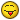Can somebody check over our work?

#### Jamstruth

##### Secondary Feline Anthropomorph
Member
sinharvest24 said:
A - B(C - D) = (E - F)/G
Attemp 1
A= (E - F)/G + B(C - D)
AG = (E - F) + B(C - D)
AG/B(C - D) = E - F
AG/B(C - D) + F = E
F = E + AG/B(C - D)

Attemp 2
A - B(C - D) = (E - F)/G.............*G
G[A-B(C-D)]=E-F
F+G[A-B(C-D)]=E
F+G(A-BC+BD)=E
F=E-G(A-BC+BD)
Attempt Pi's first, mine was way too confusing now I read it through... I've been doing too much really hard calculus and algebra lately (adv higher maths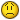)

A-B(C - D) = (E - F)/G original equation
G(A-B)(C-D) = E - F Multiply both sides by G
G(A - B)(C - D) - E = -F Take E from both sides
F = E - G(A - B)(C - D) Multiply both sides by -1
Edit: THIS IS INCORRECT!!! Spot the error and win a bowl of nothing.

The following solution IS right
A - B(C-D) = (E - F)/G
G(A - B(C-D)) = E-F Multiply both sides by G
-F = G(A-B(C-D)) - E SUbtract E from both sides
F = E - G(A-B(C-D)) Multiply both sides by -1
This is the equation's simplest form.

Unless the question specifically asks you to I wouldn't bother multiplying out any brackets you are given. If I now gave you the values of A, B, C, D, E and G then it would be a lot easier to work from this form to find F than the fully multiplied form.
Since I don't really know the level you work to your best bet is to look in your textbook and try and attempt a few questions, if you want then we'll check them.
Its a LOT easier to understand handwritten maths so if you do do this then it would be best to do it on paper and take a photo of your work to upload. We'll know the exact form of the equation you had at the start.

#### SinHarvest24

##### Shiroyasha
OP
Member
The Pi said:
C = (5 (F - 32))/9

Make F the subject.

C = (5 (F - 32))/9
9C = 5 (F - 32)
9C/5 = F - 32
9C/5 + 32 = F

is that right?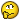@Jamstruth - i see what you did there and understand that, that is right.

#### Issac

##### Iᔕᔕᗩᑕ
Supervisor
A + B - (C(D-F(G+H))) = B + 2A/C

try that one!edit: for G

#### SinHarvest24

##### Shiroyasha
OP
Member
Issac said:
A + B - (C(D-F(G+H))) = B + 2A/C

try that one!edit: for G

A + B - (C(D-F(G+H))) = B + 2A/C

- C(D-F(G+H)) = B + 2A/C -A - B

D-F(G+H) = (B + 2A/C -A - B)/-C

-F(G+H) = (B + 2A/C -A - B)/-C - D

G+H = ((B + 2A/C -A - B)/-C - D)/-F

G = ((B + 2A/C -A - B)/-C - D)/-F - H

That's what i got....correction?

#### Jamstruth

##### Secondary Feline Anthropomorph
Member
Issac said:
A + B - (C(D-F(G+H))) = B + 2A/C

try that one!edit: for G
Do you mean B + (2A/C) or (B + 2A)/C?

Replies
2
Views
937
•Replies
15
Views
923
Replies
15
Views
1K
D
Replies
5
Views
1K
Replies
10
Views
1K

### Recent Content

7. #### [Nintendo Switch] Game Icon Customizer - simplify the method of changing game icons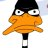@ kenenthk: Are people out there really spending \$100 on fans...